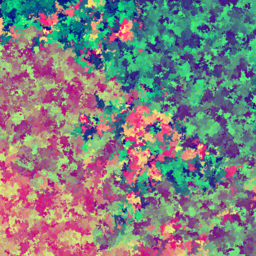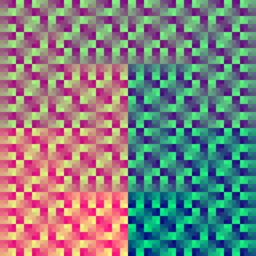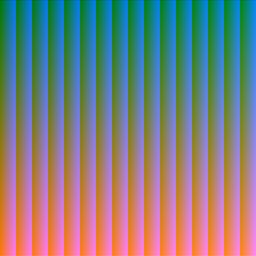# Introduction

This post just tests the CSS and code formatting on this site.

# Code & syntax highlighting

This is a test for code blocks and syntax highlighting. It's some sample code for a simple Flask route.

@app.route("/")
def index():
return "This is the homepage"

# some snippet of python


The end of the excerpt should be above.

# Text formatting

Testing Markdown and $$\KaTeX$$ formatting.

## Basic

This long paragraph should wrap nicely: lorem ipsum dolor sit amet, consectetur adipisicing elit, sed do eiusmod tempor incididunt ut labore et dolore magna aliqua. Ut enim ad minim veniam, quis nostrud exercitation ullamco laboris nisi ut aliquip ex ea commodo consequat.

You need lines of text
without a paragraph break
to write a haiku.

• Bold italic text
• Bold text
• Italic text
• More nesting
• Extra nesting
• Maximum nesting?
• Even more nesting
• Underlined text
• Crossed out text
1. Uno
2. Dos
1. Dos punto uno
2. Dos punto dos
3. Tres

## Math + KaTeX

This site uses KaTeX v0.12.0 to render $$\LaTeX$$ math formulas. Unfortunately, these math formulas won't render if JavaScript is disabled. This tests whether $$\KaTeX$$ correctly renders inline and block math.

The likelihood estimator $$L$$ of the geometric distribution ($$f(x, p) = (1-p)^{x-1} p$$):

$\gdef\p {\htmlStyle{color: #BE19FA;}{p}}$ $\gdef\Lgiven#1#2 {L\left(#1\,|\,#2\right)}$ $\gdef\Pgiven#1#2 {P\left(#1\,|\,#2\right)}$ $\Lgiven{x_1, \ldots x_{n}}{\p} = \prod_{i=1}^{n} \Pgiven{x_i}{\p} = \prod_{i=1}^{n} \left( (1-\p)^{x_i - 1} \p \right)$ Testing aligned environment: \begin{aligned} \ln{\Lgiven{x_1, \ldots x_{n}}{\p}} &= \sum_{i=1}^{n} \ln{\left((1-\p)^{x_i - 1} \p \right)}\\ &= \sum_{i=1}^{n} \left( (x_i - 1) \ln{(1-\p)} + \ln{\p} \right)\\ &= n\ln{\p} + \ln{(1-\p)} \sum_{i=1}^{n} (x_i - 1) \end{aligned}

# Blocks

## Quote blocks

Basic block quote
line 2
line 3

First paragraph of A Tale of Two Cities:

It was the best of times, it was the worst of times, it was the age of wisdom, it was the age of foolishness, it was the epoch of belief, it was the epoch of incredulity, it was the season of Light, it was the season of Darkness, it was the spring of hope, it was the winter of despair, we had everything before us, we had nothing before us, we were all going direct to Heaven, we were all going direct the other way – in short, the period was so far like the present period, that some of its noisiest authorities insisted on its being received, for good or for evil, in the superlative degree of comparison only.

## Colored blocks

This block-styled content was heavily inspired by Premonition.

I am a note

The body of the note goes here. You can write any Markdown inside the block.

Ternary Operators

This is an info block. The syntax for ternary operators in Python is a if condition else b. In Java and JavaScript, it's condition ? a : b.

StackOverflowError

Probably happening because your recursive function ends up calling itself forever. 😒

# Images, videos, & tables

Multiple images inline
Favicon image test:DFS cube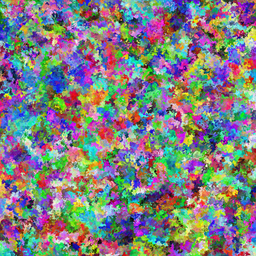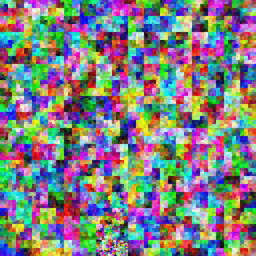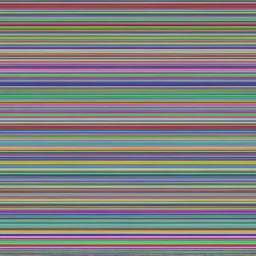Serpentine cube